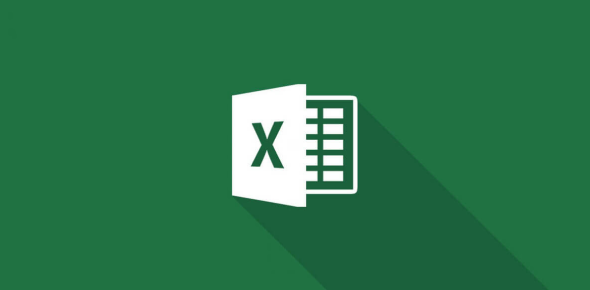# Microsoft Excel Quiz Questions And Answers

22 Questions | Total Attempts: 5640Settings.

• 1.
Is it possible to insert an image from a file into an Excel spreadsheet?
• A.

Yes

• B.

No

• 2.
Which formula can add the all the numeric values in a range of cells, ignoring those which are not numeric, and place the result in a different cell?
• A.

Count

• B.

Average

• C.

Sum

• 3.
Which of these will NOT select all the cells in a document?
• A.

Using the Edit - Select All menu item

• B.

Pressing CTRL + A on the keyboard

• C.

Clicking three times with the right mouse button in the spreadsheet

• 4.
A user wishes to remove a spreadsheet from a workbook. Which is the correct sequence of events that will do this?
• A.

Go to FILE - SAVE AS - SAVE AS TYPE - Excel 4.0 Work Sheet

• B.

Right click on the spreadsheet tab and select DELETE

• C.

Right click on the spreadsheet and select INSERT - ENTIRE COLUMN

• 5.
The cell range that starts in cell A1 and goes over to column J and down to row 15 is _____.
• A.

A1-J15

• B.

A1.J15

• C.

A1:J15

• 6.
“Month 1, Month 2, Month 3” is an example of what?
• A.

Series

• B.

Function

• C.

Formula

• D.

Syntax

• 7.
Which of the following is an absolute cell reference?
• A.

P72

• B.

G\$9

• C.

\$K356

• D.

\$F\$8

• 8.
What is the name given to a value that does NOT change when used within a formula?
• A.

Reference

• B.

Constant

• C.

Function

• D.

• 9.
What name is given to the chart background?
• A.

Background area

• B.

Chart area

• C.

Legend area

• D.

Plot area

• 10.
In which tabs would you find Chart Tools commands?
• A.

Design, Shape, Layout

• B.

Layout, Format, Type

• C.

Design, Layout, Format

• D.

Styles, Shapes, Effects

• 11.
When you have created a chart what elements of that chart can be modified?
• A.

Only the text on titles and data labels

• B.

Only the colours used within the chart

• C.

• D.

Only the data series patterns

• 12.
Every object within a chart can be individually selected for formating or editing.
• A.

True

• B.

False

• 13.
A Microsoft Excel chart can be copied into which other Microsoft Office program?
• A.

Microsoft Word

• B.

Microsoft PowerPoint

• C.

Microsoft Excel Charts can be copied into both Microsoft Word and Microsoft PowerPoint

• 14.
What would be the result of =SUM(G16,300), if G16=50?
• A.

350

• B.

250

• C.

15000

• D.

6

• 15.
What is the correct formula to calculate an average based on cells H7 to H10?
• A.

=AVE(H7:H10)

• B.

=AVERAGE(H7:H10)

• C.

=AVERAGE(H7+H8+H9+H10)/4

• D.

=AVE(H7,H8,H9,H10)/4

• 16.
What is the native file format for MS Excel?
• A.

Docb

• B.

Txtc

• C.

Xlsd

• D.

Ess

• 17.
On an Excel sheet the active cell is indicated by ___.
• A.

A dark wide border

• B.

A dotted border

• C.

• D.

None of the above

• 18.
In order to multiple items in Excel you would use:
• A.

^

• B.

@

• C.

*

• D.

#

• 19.
What program do you use to create a spreadsheet?
• 20.
When are you typing an equation into a cell the first thing that must be entered is?
• 21.
The intersection of a row and a column is called:
• 22.
Which button on the standard toolbar gives you quick access to the Sum, Average, Count, Min, and Max functions?
Related TopicsBack to top